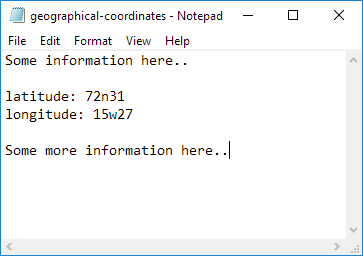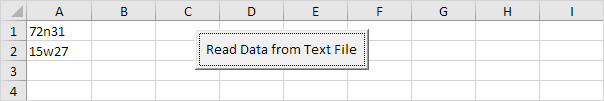Below we will look at a program in Excel VBA that reads data from a text file. This file contains some geographical coordinates we want to import into Excel.

Situation:Place a command button on your worksheet and add the following code lines:

2. We declare four variables. myFile of type String, text of type String, textline of type String, posLat of type Integer, and posLong of type Integer.

Dim myFile As String, text As String, textline As String, posLat As Integer, posLong As Integer

3. We need to initialize the variable myFile with the full path and the filename.

myFile = "C:\test\geographical-coordinates.txt"

or

use the GetOpenFilename method of the Application object to display the standard Open dialog box and select the file (without actually opening the file).

myFile = Application.GetOpenFilename()

Note: the empty part between the brackets means we give Excel VBA nothing as input. Place your cursor on GetOpenFilename in the Visual Basic Editor and click F1 for help on the arguments.

4. Add the following code line:

Open myFile For Input As #1

Note: this statement allows the file to be read. We can refer to the file as #1 during the rest of our code.

5. Add the following code lines:

Do Until EOF(1)
Line Input #1, textline
text = text & textline
Loop

Note: until the end of the file (EOF), Excel VBA reads a single line from the file and assigns it to textline. We use the & operator to concatenate (join) all the single lines and store it in the variable text.

6. Close the file.

Close #1

7. Next, we search for the position of the words latitude and longitude in the variable text. We use the Instr function.

posLat = InStr(text, "latitude")
posLong = InStr(text, "longitude")

8. We use these positions and the Mid function to extract the coordinates from the variable text and write the coordinates to cell A1 and cell A2.

Range("A1").Value = Mid(text, posLat + 10, 5)
Range("A2").Value = Mid(text, posLong + 11, 5)

9. Test the program.

Result: